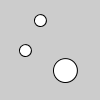Name

### PVector

Examples```v1 = PVector(40, 20)
v2 = PVector(25, 50)

ellipse(v1.x, v1.y, 12, 12)
ellipse(v2.x, v2.y, 12, 12)
ellipse(v2.x, v2.y, 24, 24)
```
Description A class to describe a two or three dimensional vector, specifically a Euclidean (also known as geometric) vector. A vector is an entity that has both magnitude and direction. The datatype, however, stores the components of the vector (x,y for 2D, and x,y,z for 3D). The magnitude and direction can be accessed via the methods mag() and heading().

In many of the Processing examples, you will see PVector used to describe a position, velocity, or acceleration. For example, if you consider a rectangle moving across the screen, at any given instant it has a position (a vector that points from the origin to its location), a velocity (the rate at which the object's position changes per time unit, expressed as a vector), and acceleration (the rate at which the object's velocity changes per time unit, expressed as a vector).

Since vectors represent groupings of values, we'll need to do some "vector" math, which is made easy by the methods inside the PVector class, or, in Python Mode, in some cases the traditional mathematical operators have been "overloaded" to work with PVectors: "+" adds vectors, "-" subtracts vectors, "*" does scalar multiplication, and "/" does scalar division. The related augmented assigment operators, "+=", "-=", "*=", and "/=", also work.
Syntax
```	v = PVector(x, y)
v = PVector(x, y, z)
```
Parameters
x int: x component of the PVector int: y component of the PVector int: z component of the PVector
Methods
set() Set the components of the vector Make a new 2D unit vector with a random direction. Make a new 3D unit vector with a random direction. Make a new 2D unit vector from an angle Make a new 2D unit vector from an angle Get a copy of the vector Calculate the magnitude of the vector Calculate the magnitude of the vector, squared Adds x, y, and z components to a vector, one vector to another, or two independent vectors Subtract x, y, and z components from a vector, one vector from another, or two independent vectors Multiply a vector by a scalar Divide a vector by a scalar Calculate the distance between two points Calculate the dot product of two vectors Calculate and return the cross product Normalize the vector to a length of 1 Limit the magnitude of the vector Set the magnitude of the vector Calculate the angle of rotation for this vector Rotate the vector by an angle (2D only) Linear interpolate the vector to another vector Calculate and return the angle between two vectors Return a representation of the vector as a float array

Updated on Mon Sep 21 15:53:25 2020.

If you see any errors or have comments, please let us know.